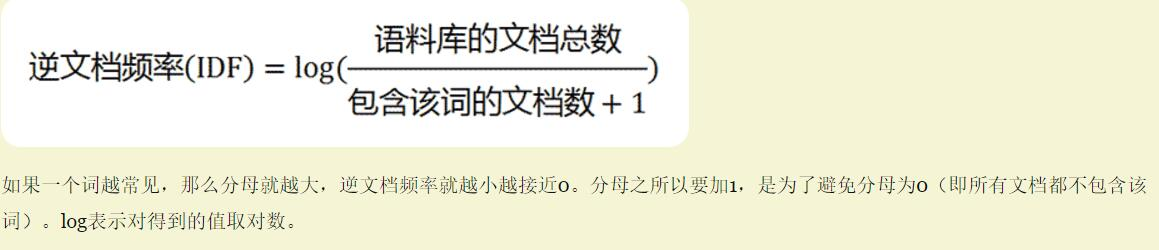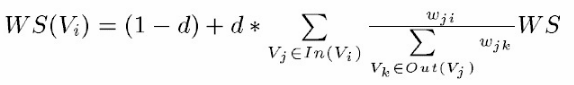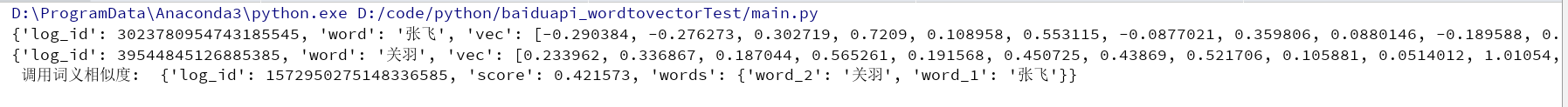# 简单NLP分析套路（2）----分词，词频，命名实体识别与关键词抽取

google 近期发布了颠覆性的NLP模型–BERT ,大家有空可以了解一下，

https://mp.weixin.qq.com/s/EPEsVzbkOdz9GovrAM-p7g

• 分词
• 词频
• 命名实体识别
• 关键词抽取

# 中文分词技术

## 评测参考

https://blog.csdn.net/riario/article/details/78259877

## 哈工大语言云 ltp

LTP_DATA_DIR =  r'..\ltp_data_v3.4.0'  # ltp模型目录的路径
cws_model_path = os.path.join(LTP_DATA_DIR, 'cws.model')  # 分词模型路径，模型名称为cws.model
pos_model_path = os.path.join(LTP_DATA_DIR, 'pos.model')  # 词性标注模型路径，模型名称为pos.model
ner_model_path = os.path.join(LTP_DATA_DIR, 'ner.model')  # 命名实体识别模型路径，模型名称为pos.model
par_model_path = os.path.join(LTP_DATA_DIR, 'parser.model')  # 依存句法分析模型路径，模型名称为parser.model
srl_model_path = os.path.join(LTP_DATA_DIR, 'pisrl_win.model')  # 语义角色标注模型目录路径，注意windows 和linux 使用不同模型

def main():
words = segmentor('我家在中科院，我现在在北京上学。中秋节你是否会想到李白？')

print(roles)

# 分句，也就是将一片文本分割为独立的句子
def sentence_splitter(sentence='你好，你觉得这个例子从哪里来的？当然还是直接复制官方文档，然后改了下这里得到的。我的微博是MebiuW，转载请注明来自MebiuW！'):
sents = SentenceSplitter.split(sentence)  # 分句
print('\n'.join(sents))

"""分词"""
def segmentor(sentence=None):
segmentor = Segmentor()  # 初始化实例
segmentor.load(cws_model_path)  # 加载模型
words = segmentor.segment(sentence)  # 分词
#默认可以这样输出
print ('\t'.join(words))
# 可以转换成List 输出
words_list = list(words)
segmentor.release()  # 释放模型
return words_list


https://blog.csdn.net/weixin_40899194/article/details/79702468

## 基于深度学习方法的中文分词

https://github.com/rockyzhengwu/FoolNLTK

## 一个领域细分的中文分词工具包（北大最新开源）

https://github.com/lancopku/PKUSeg-python

# 信息检索与关键词提取

## TF-IDF

TF-IDF原理概述

TF-IDF不但考虑了一个词出现的频率TF，也考虑了这个词在其他文档中不出现的逆频率IDF，很好的表现出了特征词的区分度，是信息检索领域中广泛使用的一种检索方法。

Tf-idf算法公式以及说明:def getTopkeyWordsTFIDF(stop_word_file_path,topK=100,content = ''):
try:
jieba.analyse.set_stop_words(stop_word_file_path)
tags = jieba.analyse.extract_tags(content, topK, withWeight=True,allowPOS=('ns', 'n', 'vn', 'v'))
for v, n in tags:
print (v + '\t' + str((n )))
top_word_dict_TFIDF[v] = n * 100
#tfidf *100 作为词频
except Exception as e:
print(e)
finally:
pass

'''

'''


## TEXTRANK

TextRank 算法是一种用于文本的基于图的排序算法。其基本思想来源于谷歌的 PageRank算法, 通过把文本分割成若干组成单元(单词、句子)并建立图模型, 利用投票机制对文本中的重要成分进行排序, 仅利用单篇文档本身的信息即可实现关键词提取、文摘。和 LDA、HMM 等模型不同, TextRank不需要事先对多篇文档进行学习训练, 因其简洁有效而得到广泛应用。

TextRank 一般模型可以表示为一个有向有权图 G =(V, E), 由点集合 V和边集合 E 组成, E 是V ×V的子集。图中任两点 Vi , Vj 之间边的权重为 wji , 对于一个给定的点 Vi, In(Vi) 为 指 向 该 点 的 点 集 合 , Out(Vi) 为点 Vi 指向的点集合。点 Vi 的得分定义如下:textRank认为一个节点如果入度多且权重大，那么这个节点越重要。

def getTopkeyWordsTextRank(stop_word_file_path, topK=100, content=''):
try:
jieba.analyse.set_stop_words(stop_word_file_path)

tags = jieba.analyse.textrank(content, topK, withWeight=True, allowPOS=('ns', 'n', 'vn', 'v'))
for v, n in tags:
print(v + '\t' + str(((n))))
top_word_dict_TEXTRANK[v] = n * 100
# tfidf *100 作为词频
except Exception as e:
print(e)
finally:
pass

'''

'''


# word2vector

https://blog.csdn.net/wangyaninglm/article/details/81232724


# -*- coding:utf-8 -*-
"""@author:season@file:main.py@time:2018/6/1323:01"""

from aip import AipNlp

""" 你的 APPID AK SK """
APP_ID = ''
API_KEY = ''
SECRET_KEY = ''

client = AipNlp(APP_ID, API_KEY, SECRET_KEY)

word1 = "张飞"
dict_zhangfei = {}
word2 = "关羽"
dict_liubei = {}

""" 调用词向量表示 """
dict_zhangfei = client.wordEmbedding(word1)
print(dict_zhangfei)
dict_liubei = client.wordEmbedding(word2)
print(dict_liubei)

vector_zhangfei = dict_zhangfei['vec']
vector_liubei = dict_liubei['vec']

import numpy as np
import math
def Cosine(vec1, vec2):
npvec1, npvec2 = np.array(vec1), np.array(vec2)
return npvec1.dot(npvec2)/(math.sqrt((npvec1**2).sum()) * math.sqrt((npvec2**2).sum()))
# Cosine，余弦夹角

print(""" 调用词义相似度: """,client.wordSimEmbedding(word1, word2))
print("余弦相似度：",Cosine(vector_zhangfei, vector_liubei))# 未完待续

NLP系列文章: